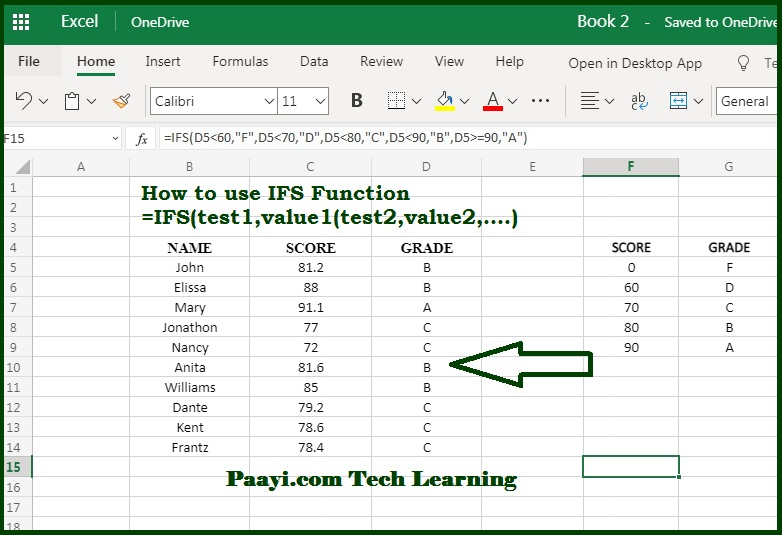# Learn How to Use Microsoft Excel IFS Function

Written by | 0 Comments | 691 Views

In this article, you will learn how to use the Microsoft Excel IFS function and its prime function in Microsoft Excel. You will also get to know the Microsoft Excel IFS function return value and syntax with the help of some examples.

## Microsoft Excel IFS Function

The IFS function is used to test the multiple conditions and returns first true. That implies, the IFS Microsoft Excel function runs numerous tests and returns the value according to the first TRUE result. You can use the IFS function without multiple nests like IF and can evaluate the numerous conditions. IFS allows formulas that are shorter, easier, and easy to pursue.

IFS Functions Return Value

The value is related to the first TRUE result.

IFS Function Syntax

=IFS(test1,value1, [test2,value2,...)

Where:

• test1: First logical result.
• value1: Result when test1 is TRUE.
• test2: Second logical result (Optional)
• value2: Result when test2 is TRUE (Optional)

## How to Use Microsoft Excel IFS Function?IFS is a new function and available in Office 365 and Excel 2019. As we know, you can use the IFS function to test the multiple conditions and return the value corresponding to the first TRUE result. You can use the IFS function without nesting to test the multiple conditions, unlike the IF function. Formulas based on the IFS function are easy to read and shorter.

The conditions are entered in test/value pairs, and each test represents a logical test that returns the value TRUE or FALSE corresponding to it. The IFS formula with four values will be given below:

=IFS(

test1,value1

test2,value2

test3,value3

test4,value4

)

The first test that returns the TRUE value will be the winner.